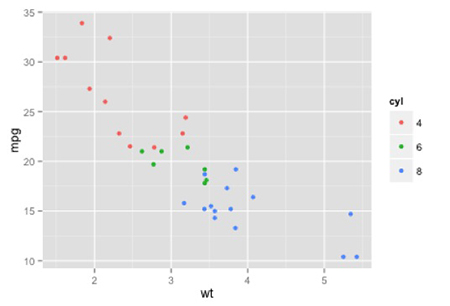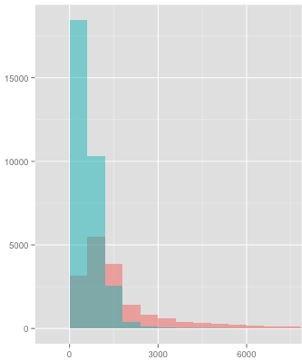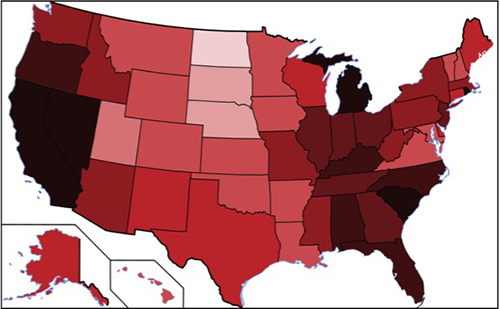15.071 | Spring 2017 | Graduate

# The Analytics Edge

7.2 Visualizing the World: An Introduction to Visualization

## 7.2 Visualizing the World: An Introduction to Visualization

### Quick Question

In this quick question, we’ll be asking you questions about the following three plots, that we saw in Video 1. We’ll refer to them as the “Scatterplot”, the “Histogram”, and the “US Map”.

The Scatterplot:The Histogram:The US Map:In the Scatterplot, what are the geometric objects?

Bars  close
Points  check
Lines  close
Polygons  close
Boxes  close

Explanation The geometric objects for the Scatterplot are points, for the Histogram are bars, and for the US Map are polygons (the States). All three plots defined colors in the plot.

In the Histogram, what are the geometric objects?

Bars  check
Points  close
Lines  close
Polygons  close
Boxes  close

Explanation The geometric objects for the Scatterplot are points, for the Histogram are bars, and for the US Map are polygons (the States). All three plots defined colors in the plot.

In the US Map, what are the geometric objects?

Bars  close
Points  close
Lines  close
Polygons  check
Boxes  close

Explanation The geometric objects for the Scatterplot are points, for the Histogram are bars, and for the US Map are polygons (the States). All three plots defined colors in the plot.

All three of these plots defined a particular aesthetic property. What is it?

The x-axis label.  close
The y-axis label.  close
Different shapes for different types of observations.  close
Colors.  check
A legend.  close

Explanation The geometric objects for the Scatterplot are points, for the Histogram are bars, and for the US Map are polygons (the States). All three plots defined colors in the plot.

## Course Info

Spring 2017
##### Learning Resource Types
Lecture Videos
Lecture Notes
Problem Sets with Solutions